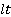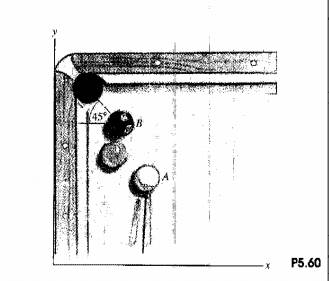### Create an Account

Already have account?

### Forgot Your Password ?

Home / Questions / In Problem 560 what are the velocity vectors of the two P5S8 balls just after the impact i...

# In Problem 560 what are the velocity vectors of the two P5S8 balls just after the impact if the coefficient restitution is

In Problem 5.60, what are the velocity vectors of the two P5.S8 balls just after the impact if the coefficient restitution is e = 0,9&#39;1

Problem 5.60

The cue gives the cue ball A ~ velocity parallel to the y axis.hits the 8-ball B and knocks it straight I to the comer pocket. If the magnitude of the velocity of the&#39; cue ball just before the impact is 2 m/s and the coefficient of restitution is e = 1, what are the velocity vectors the two balls just&#39; after the impact? (The balls are of equal mass.)Jun 16 2020 View more View Less

#### Answer (Solved)Subscribe To Get Solution